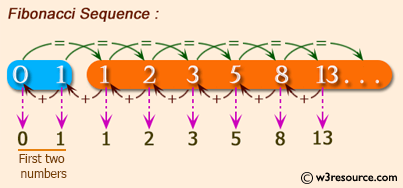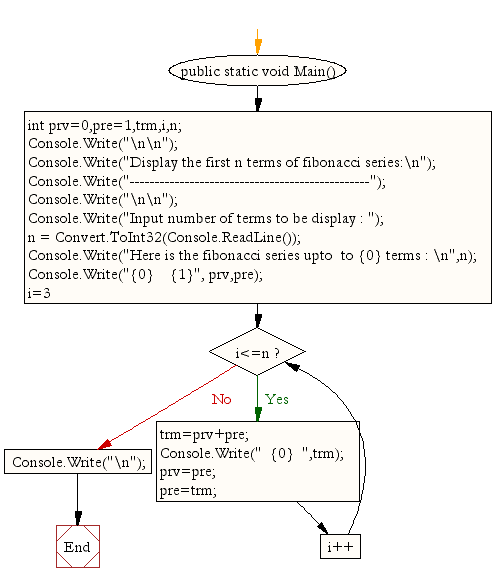﻿ C# - Display the first n terms of fibonacci series# C# Sharp Exercises: Display the first n terms of Fibonacci series

## C# Sharp For Loop: Exercise-35 with Solution

Write a program in C# Sharp to display the first n terms of Fibonacci series.
The series is as follows :
Fibonacci series 0 1 2 3 5 8 13 .....

Pictorial Presentation:Sample Solution:

C# Sharp Code:

``````using System;
public class Exercise35
{
public static void Main()
{
int prv=0,pre=1,trm,i,n;

Console.Write("\n\n");
Console.Write("Display the first n terms of fibonacci series:\n");
Console.Write("------------------------------------------------");
Console.Write("\n\n");

Console.Write("Input number of terms to be display : ");
Console.Write("Here is the fibonacci series upto  to {0} terms : \n",n);
Console.Write("{0}    {1}", prv,pre);

for(i=3;i<=n;i++)
{
trm=prv+pre;
Console.Write("  {0}  ",trm);
prv=pre;
pre=trm;
}
Console.Write("\n");
}
}
```
```

Sample Output:

```Display the first n terms of fibonacci series:
------------------------------------------------
Input number of terms to be display : 10
Here is the fibonacci series upto  to 10 terms :
0    1  1    2    3    5    8    13    21    34
```

Flowchart:C# Sharp Code Editor:

Contribute your code and comments through Disqus.

What is the difficulty level of this exercise?

Test your Programming skills with w3resource's quiz.

﻿